# The Discontinuous Groups of Rotation and Translation in the Plane

## Group Elements and Binary Operation

The elements in our group are rotations and translations, written as r[{x,y},α] and t[{x,y}], where the {x,y} is the center of rotation or a vector specifying a translation. Two elements are considered identical if their actions on the plane are identical. For example, r[{c1,c2},π] == r[{c1,c2}, -π] and r[{x,y},0] == t[{0,0}] == Identity. We will sometimes call the elements in our group symmetry operations.

We define our binary operation to be the succession of symmetry operations. Since the combination of translation and rotation are equivalent to a unique translation or rotation, thus our binary operation is well defined.

It is obvious that closure, identity, inverse, and associativity are satisfied. For associativity, suppose A, B, C are three symmetry operations. Associativity axiom states that (A*B)*C==A*(B*C). This is satisfied because in both sides of the equation, the symmetry operations are performed in the sequence A, B, C. For the case of A*(B*C), it merely means that we combine the operation B and C into one, and perform A followed by (B*C). In particular, A*(B*C) does not mean (B*C)*A. It is also obvious to see that our groups are not communitive, because in general a rotation followed by a translation is not the same if order is reversed.

In forming our group with rotation and translation, we further require that the group satisfy a property. Suppose we apply all symmetry operations in our group to a fixed point. We require that in a finite region there are only finitely many distinct points so obtained. Transformation groups that satisfy this requirement are called discontinuous. In summary, we are restricting our investigation to discontinuous group of rotations and translations in the plane. We set out to find all such groups.

Note that the study of plane symmety is a study of structure. Structure is a algebraic notion and has nothing to do with angles or distances. Do not get confused by elements in our group with points, angles, or distances in the plane. The investigation of symmetry — the structure that we are trying to extract from the plane — is based on operations (rotations, translations) that involve concepts of points, distances and angles. This is why we do talk about distances and angles in discussion of symmetry. Analogy: Car speed analysis (maximum speed, minimum speed, acceleration rate,…etc.) is not about distance and time, but such notions are not avoidable.

We will denote groups using set notation, with curly braces. For example, {r[{0,0},0],r[{0,0},π]} is a group consisting two rotations with 0 and π angles, centered on origin. More often we'll need set-bulding notation. For example, {t[{n,0}] | n is a integer} is the same as {t[{0,0}],t[{-1,0}],t[{1,0}],t[{-2,0}],t[{2,0}],…}. For clarity, we abbreviate it into just {t[{n,0}]}. Another example: {r[P,2*π/3*n]} is a cyclic group of order 3.

## Isomorphism and Representation

Consider following groups {t[{n,0}]}, {t[{n,1}]}, {t[{2*n,0}]}, and {t[{-n,0}]} where n is a integer. These groups are all isomorphic to the cyclic group of infinite order. Be aware that we are interested in distinct group with respect to isomorphism, not representation. Another example: the group {r[{0,0},α*n]} is isomorphic to {r[P,α*n]} for any fixed point P.

Suppose we have a group {Identity, r[{x1,y1},α],…, t[{x2,y2}],…}. In general, if every base point of our group elements (symmetry operations) are transformed by a rigid motion (or dilation/contraction), the resulting group is isomorphic to the original. For the sake of uniformness, whenever possible, we'll represent our group by a isomorphic one that contains a rotation centered on the origin, and the translation with shortest length is t[{1,0}]. (If a group contains both rotation and translation, it is possible to have such isomorphic group. The proof should be easy after reading.)

## Visual Representation

Later on we'll have illustrations for our groups, thus here we discuss issues of graphics representation of groups. This section is not required in our derivation process, and can be skipped if you are a purist.

Suppose P and Q are nonparallel vectors. The set {n*P+m*Q} is called a plane lattice. (m, n are integers.) Given a lattice, there are many pairs of vectors that can generate it. We can select a standard pair by requiring that the shorter one lies on the x-axes and the second shortest one in the first quadrant. The standard basis vectors of a lattice forms a reduced parallelogram of the lattice.

Lattices are classified by their symmetry into the following five types:

1. Triangular (aka hexagonal): The standard basis vectors are equal in length and form a angle of 2*π/6.
2. Square: The standard basis vectors are equal in length and form a angle of 2*π/4.
3. Rhombic (aka centered cell): The standard basis vectors are equal in length, and forming a angle that's not 2*π/6 or 2*π/4.
4. Rectangular: The standard basis vectors are not equal in length but form a angle of 2*π/4.
5. Parallelogram: Any lattice that's not one of the above. i.e. standard basis vectors not equal in length and forms a angle that's not one of 2*π/6 or 2*π/4.Five types of lattice. Triangular, Square, Rhombic (rotated), Rectangular, and Parallelogram.

Two points are said to be equivalent under a given group if there is a element in the group that transforms one point to the other. A unit cell is a connected region of a wallpaper such that the whole wallpaper can be generated by applying translations to that region. A fundamental region is a smallest region in a wallpaper such that the whole wallpaper can be generated by applying symmetries (rotation, reflection, translation) to that region. Fundamental region can be chosen so it's a part of a given unit cell, or equal to it. Note that both unit cell and fundamental region are not unique. Other authors use different terms for either: primitive cell, fundamental cell, lattice unit, etc. (More formally, a unit cell can be defined as any connected region that does not contain a pair of equivalent points in its interior but that cannot be enlarged without losing this property. [David Hilbert, S. Cohn-Vossen])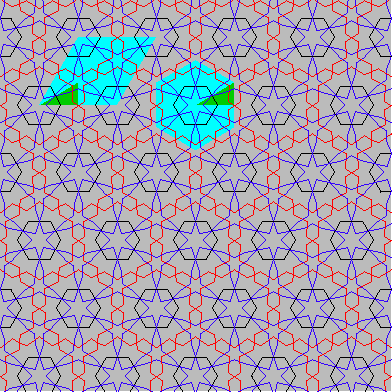The skyblue regions are examples of two unit cells. The green regions are examples of two fundamental regions.

As we will see, the translations in a group forms a lattice, and so do the centers of rotations. The translation lattice is a subset of the rotation lattice, not necessarily a proper subset. Our groups can be represented visually using symbols fixed on the lattice. Regular n-gon represents n-fold rotation elements. (two-fold rotation are represented by a rhombus) Two arrows represent the basis vectors of the translation lattice. Thus, a visual representation of a group consists of a lattice of rotation symbols plus a pair of floating arrows indicating the translation lattice. “Floating” because there is no positional relation between the translation lattice and the rotation lattice, but there is a scale and orientation relation, thus it is necessary to indicate the translation axes.

 2-fold center of rotation3-fold center of rotation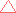4-fold center of rotation6-fold center of rotation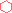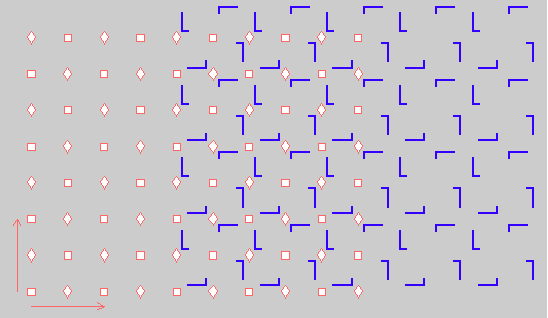The group 442 (p4) represented by its symmetry element on the left, and by a wallpaper on the right.

Another method of visual representation is by applying all elements in the group to a motif in the plane. The motif can be small or as large to fill out the whole plane. We use a non-symmetric motif to avoid generating images that can represent more than one group. This method has a direct two-way relation: the graphics represents the group and the graphics' symmetry is represented by the group. We will use a L-shaped line segment as our motif, having coordinates Line[{{2/12,1/12},{5/12,1/12},{5/12,2/12}}]. This almost gives us a unique graphics for each group. (“almost” because some groups do not have a fixed lattice. For example, the group 2222 (p2) can be based on any parallelogram)

A third method to represent our groups visually is by Cayley diagrams (digraphs). Each element is a node and directed edges represent generators. We'll not use it here.

## Theorems on Group Elements

Here are some theorems of the nature that certain elements in the group imply other elements. These will be used later when we derive groups. Theorem names are give for identification purposes.

### Theorem: Lattice of Translations

If t[P] is a element, then t[P*n] is a element, where P is a vector and n is a integer. (because t[P*n]==t[P]^n, the right hand side is guaranteed by group axioms)

If t[P] and t[Q] are elements where P and Q are not parallel, then t[P*m+Q*n] are elements where m and n are integers (because t[P*m+Q*n]==t[P]^m*t[Q]^n). Two non-parallel translations imply a lattice of translations.

### Theorem: Transitivity of Rotation

If r[P,α] is a element, and if m is a element that maps P to Q, then r[Q,α] is also a element of the group. In symbols, we have

• (r[P,α] and t[V]) implies r[P+V,α]
• (r[P,α] and r[C,β]) implies r[r[C,β][P],α]

Proof: let r1:=r[P,α], and let m be the motion that maps P to Q. We show that m^-1*r1*m==r[Q,α].

• (1) In m^-1*r1*m, Q is a fixed point because m^-1 moves a point at Q to location of P, r1 doesn't move point at location P, then m moves point in location P back to location Q. Existence of a fixed point implies a rotation or identity.
• (2) By the rotation angle addictivity theorem, the angle in m^-1*r1*m must be α, because angles in m^-1 and m cancels out. Now m^-1*r1*m has a fixed point Q, and has angle α, thus it is r[Q,α].

End of proof.

As a consequence, a lattice {t[A*m+B*n]} and a rotation r[P,α] guarantees a lattice of rotations {r[P+(A*m+B*n),α]}.

### Theorem: Transitivity of Translation

If t[P] and r[C,α] are elements, then t[Q] is a element where Q makes a angle α with P. (Proof synopsis: t[Q]==r^-1*t*r where r:=r[C,α], t:=t[P], and Q:=r[{0,0},α][P].)

Proof: Let r1:=r[C,α], t1:=t[P], and Q:=r[{0,0},α][P]. We show that t[Q]==r1^-1*t1*r1. By addictivity of rotation angle theorem, r1^-1*t1*r1 have angle zero, thus it is a translation or identity. Now look at the action r^1-1*t1*r1 on C. r1^-1 does not move it. t1*r1 moves it to r[C,α][C+P]. Thus r1^-1*t1*r1 maps C to r[C,α][C+P], thus r1^-1*t1*r1 is not identity, and thus must be a translation, with the vector Q:=(r[C,α][C+P]-C). By elementary theorem of vector property, the point (r[C,α][C+P]-C) is just P rotated by α.

### Theorem: 2-Fold Rotations on Midpoints of Lattice

If t[P] and r[C,2*π/2] are elements in a group, then r[C+P/2,2*π/2] is also in the group. (Proof synopsis: r[C+P/2,2*π/2]==r[C,2*π/2]*t[P])

Proof: Let's look at the action of r[C,2*π/2]*t[P] on points C and D:=C+P. It is obvious that C':=D and D':=C. Thus it is a rotation on the midpoint of C and D, we have r[C,2*π/2]*t[P]==r[(C+(C+P))/2,2*π/2]==r[C+P/2,2*π/2]. End of proof.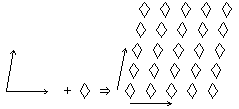A translation lattice and a two-fold rotation implies a two-fold rotation lattice of half scale.

Together with the transitivity of rotations theorem, we have the general result that a lattice {t[P*m+Q*n]} and a 2-fold rotation r[C,2*π/2] guarantees a lattice of 2-fold rotations {r[C+(P*m+Q*n),2*π/2]} and 2-fold rotations on all midpoints of the lattice {r[C+(P*m+Q*n)/2,2*π/2]}.

### Theorem: Distinct Rotations Guarantees a Translation

If a group contains two rotations on two distinct points, then it contains a translation.

Proof: Let r1:=r[A1,a1], r2:=r[A2,a2] where A1 and A2 are distinct centers and a1 > a2 > 0. If one angle is a integer m multiple of the other, than r1*r2^-m is a translation by theorem of addictivity of rotation angle. Now suppose a2*m ≠ a1 for all m. r1*r2^-n implies a rotation with angle a3 where a3 is less than a2, and with center distinct from its parents. If a3*m==a2 for some integer m, then r2*r3^-m is a translation, else, repeating the process we get a angle a[n] that is smaller than its predecessors. We must reach a point where a[n]*m==a[n-1] because a discontinuous group cannot have rotation angles that's infinitly small.

### Definition: n-fold rotation

Suppose r[P,α] is a element. We have r^n==Identity, where n is a integer, otherwise the group wouldn't be discontinuous. Therefore, the rotation angle for all discontinuous groups must be a integer multiple of 2*π/n, where n is a fixed positive integer. When we say a point P has n-fold rotation, we mean r[P,2*π/n] is a element in the group under discussion.

### Theorem: Crystallographic Restriction

If a group contain rotations on at least two distinct centers (i.e. excluding the cyclic group {r[P,2*π/n*m]} with a single rotation center P.), then its rotations must be one of 2, 3, 4, or 6-fold. That is, the least positive angles of rotation must be one of 2*π/n, where n is 2, 3, 4, or 6.

Proof: We know by previous theorem that two distinct rotation centers gurantee a translation. Suppose we have a rotation r[A,α] and pick a translation t[V] with the least length. By theorem transitivity of rotation, we also have a rotation r[B,α] where B:=A+V. Again, By theorem transitivity of rotation on rotation A and B, we have r[C,α] where C:=r[B,α][A], and again we have r[D,α] where D:= r[C,α][B]. In summary, we have:

• r[A,α]. (given)
• t[V], where V is the least length. (Implied)
• r[B,α], B:=t[V][A]. (b==t[V]^-1*r[A,α]*t[V])
• r[C,α], C:=r[B,α][A]. (r[A,α]==r[B,α]^-1*r[A,α]*r[B,α])
• r[D,α], D:=r[C,α][B]. (r[B,α]==r[C,α]^-1*r[B,α]*r[C,α])A, B, C, D forms a quadrilateral with three equal sides AB, BC, CD and angles ABC==BCD==α.

So now we have four rotations on A,B,C,D forming a quadrilateral with three equal sides AB, BC, CD and angles ABC==BCD==α.

By transitivity of translation on t[V] and r[B,α], we have t[B-C]. Further, we have t[B-C]^-1*t[V]== t[C-B]*t[B-A]==t[C-A]. Similar argument also guarantees t[D-C] and t[D-A]. In summary we have all translations going from any of A,B,C,D to any other.

If α < 2*π/6 or α == 2*π/5, then length of t[D-A] is smaller than t[B-A]==t[V], contradicting that t[V] is chosen to have the smallest length. End of Proof.